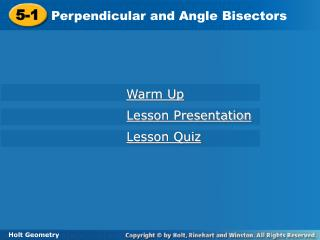DownloadDownload Presentation5-1

# 5-1

Télécharger la présentation## 5-1

- - - - - - - - - - - - - - - - - - - - - - - - - - - E N D - - - - - - - - - - - - - - - - - - - - - - - - - - -
##### Presentation Transcript

1. 5-1 Perpendicular and Angle Bisectors Warm Up Lesson Presentation Lesson Quiz Holt Geometry

2. Warm Up Construct each of the following. 1.A perpendicular bisector. 2. An angle bisector. 3. Find the midpoint and slope of the segment (2, 8) and (–4, 6).

3. Objective SWBAT prove and apply theorems about angle bisectors. HW Page 304 {5, 6, 7, 8, 15, 17, 19, 31}

4. Vocabulary equidistant locus

5. When a point is the same distance from two or more objects, the point is said to be equidistantfrom the objects. Triangle congruence theorems can be used to prove theorems about equidistant points.

6. A locus is a set of points that satisfies a given condition. The perpendicular bisector of a segment can be defined as the locus of points in a plane that are equidistant from the endpoints of the segment.

7. Remember that the distance between a point and a line is the length of the perpendicular segment from the point to the line.

8. Based on these theorems, an angle bisector can be defined as the locus of all points in the interior of the angle that are equidistant from the sides of the angle.

9. Example 2A: Applying the Angle Bisector Theorem Find the measure. BC BC = DC  Bisector Thm. BC = 7.2 Substitute 7.2 for DC.

10. Since EH = GH, and , bisects EFGby the Converse of the Angle Bisector Theorem. Example 2B: Applying the Angle Bisector Theorem Find the measure. mEFH, given that mEFG = 50°. Def. of  bisector Substitute 50° for mEFG.

11. Since, JM = LM, and , bisects JKL by the Converse of the Angle Bisector Theorem. Example 2C: Applying the Angle Bisector Theorem Find mMKL. mMKL = mJKM Def. of  bisector 3a + 20 = 2a + 26 Substitute the given values. a + 20 = 26 Subtract 2a from both sides. a= 6 Subtract 20 from both sides. So mMKL = [2(6) + 26]° = 38°

12. Check It Out! Example 2a Given that YW bisects XYZ and WZ = 3.05, find WX. WX = WZ  Bisector Thm. WX = 3.05 Substitute 3.05 for WZ. So WX = 3.05

13. Check It Out! Example 2b Given that mWYZ = 63°, XW = 5.7, and ZW = 5.7, find mXYZ. mWYZ + mWYX = mXYZ  Bisector Thm. mWYZ = mWYX Substitute m WYZ for mWYX . mWYZ +mWYZ= mXYZ Simplify. 2mWYZ = mXYZ 2(63°) = mXYZ Substitute 63° for mWYZ . 126° = mXYZ Simplfiy.

14. John wants to hang a spotlight along the back of a display case. Wires AD and CD are the same length, and A and C are equidistant from B. How do the wires keep the spotlight centered? It is given that . So D is on the perpendicular bisector of by the Converse of the Angle Bisector Theorem. Since B is the midpoint of , is the perpendicular bisector of . Therefore the spotlight remains centered under the mounting. Example 3: Application

15. S is equidistant from each pair of suspension lines. What can you conclude about QS? QS bisects PQR. Check It Out! Example 3

16. Use the diagram for Items 3–4. 3. Given that FH is the perpendicular bisector of EG, EF = 4y – 3, and FG = 6y – 37, find FG. 4. Given that EF = 10.6, EH = 4.3, and FG = 10.6, find EG. Lesson Quiz: Part I Use the diagram for Items 1–2. 1. Given that mABD = 16°, find mABC. 2. Given that mABD = (2x + 12)° and mCBD = (6x – 18)°, find mABC. 32° 54° 65 8.6

17. Lesson Quiz: Part II 5. Write an equation in point-slope form for the perpendicular bisector of the segment with endpoints X(7, 9) and Y(–3, 5) .